Creating a map with contour lines

Plotting a contour map is handled by pygmt.Figure.grdcontour.

import pygmt

# Load sample earth relief data
grid = pygmt.datasets.load_earth_relief(resolution="05m", region=[-92.5, -82.5, -3, 7])

Create contour plot

The pygmt.Figure.grdcontour method takes the grid input. It plots annotated contour lines, which are thicker and have the elevation/depth written on them, and unannotated contour lines. In the example below, the default contour line intervals are 500 meters, with an annotated contour line every 1000 meters. By default, it plots the map with the equidistant cylindrical projection and with no frame.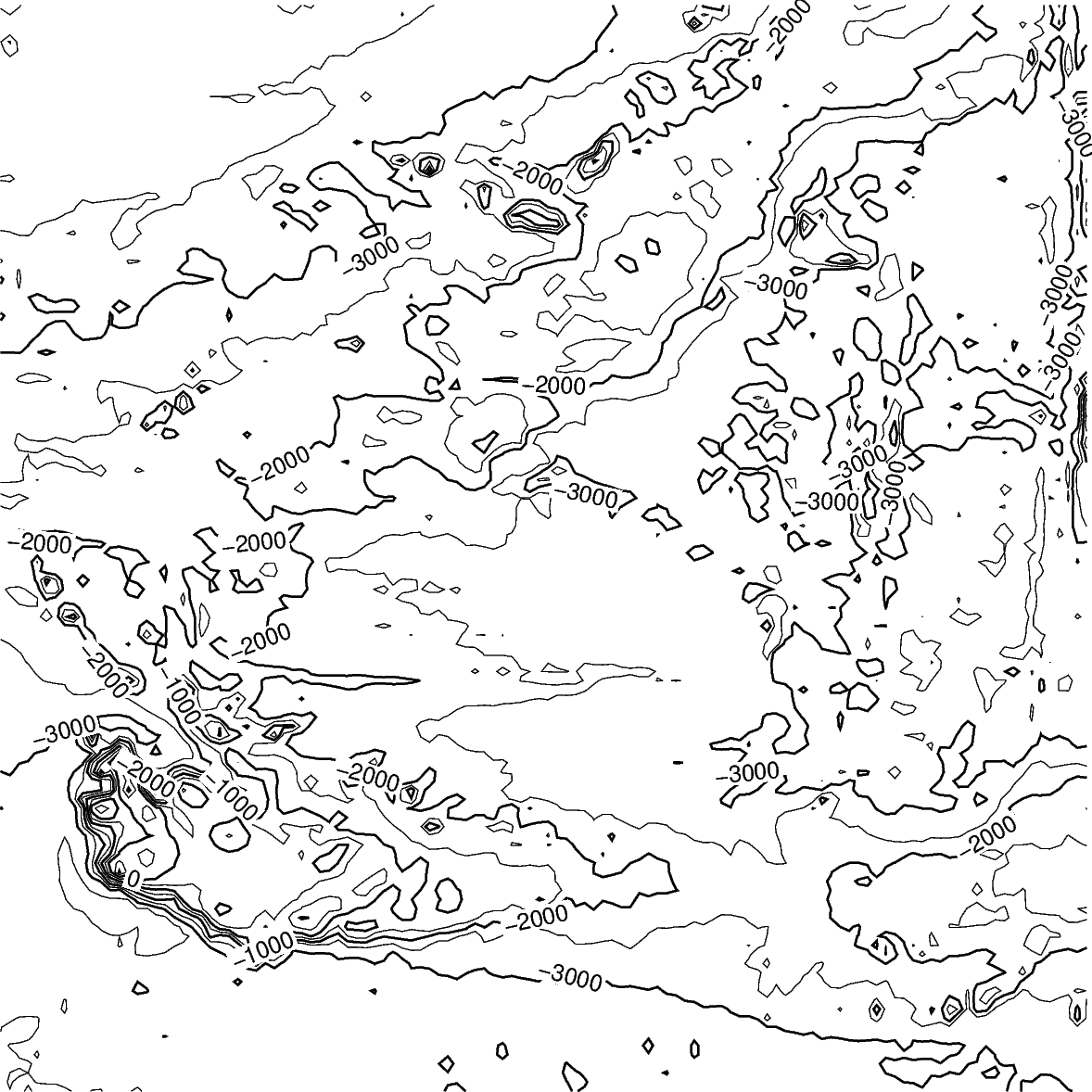Out:

<IPython.core.display.Image object>

Contour line settings

Use the annotation and interval arguments to adjust contour line intervals. In the example below, there are contour intervals every 250 meters and annotated contour lines every 1,000 meters.

fig = pygmt.Figure()
fig.grdcontour(
annotation=1000,
interval=250,
grid=grid,
)
fig.show()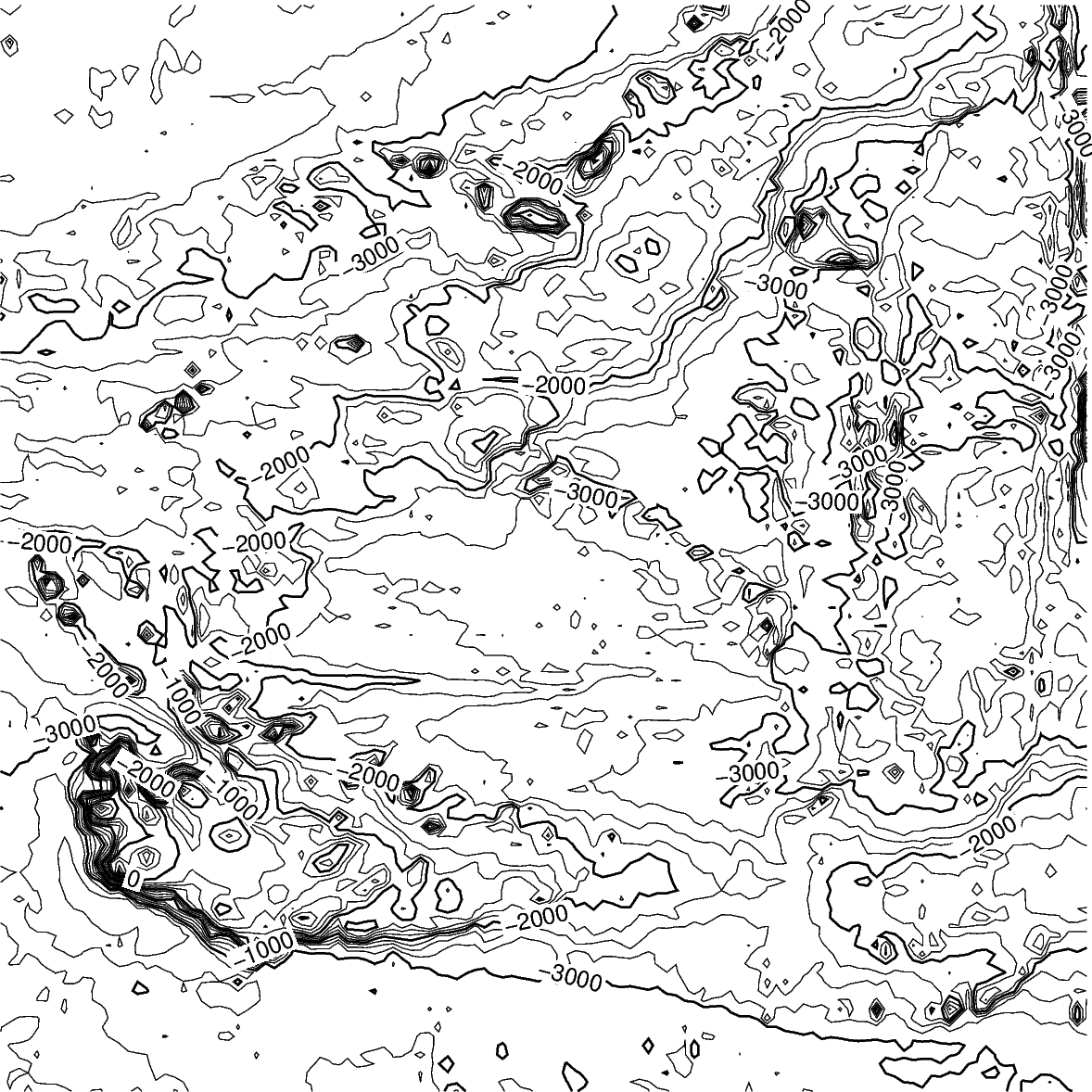Out:

<IPython.core.display.Image object>

Contour limits

The limit argument sets the minimum and maximum values for the contour lines. The argument takes the low and high values, and is either a list (as below) or a string limit="-4000/-2000".

fig = pygmt.Figure()
fig.grdcontour(
annotation=1000,
interval=250,
grid=grid,
limit=[-4000, -2000],
)
fig.show()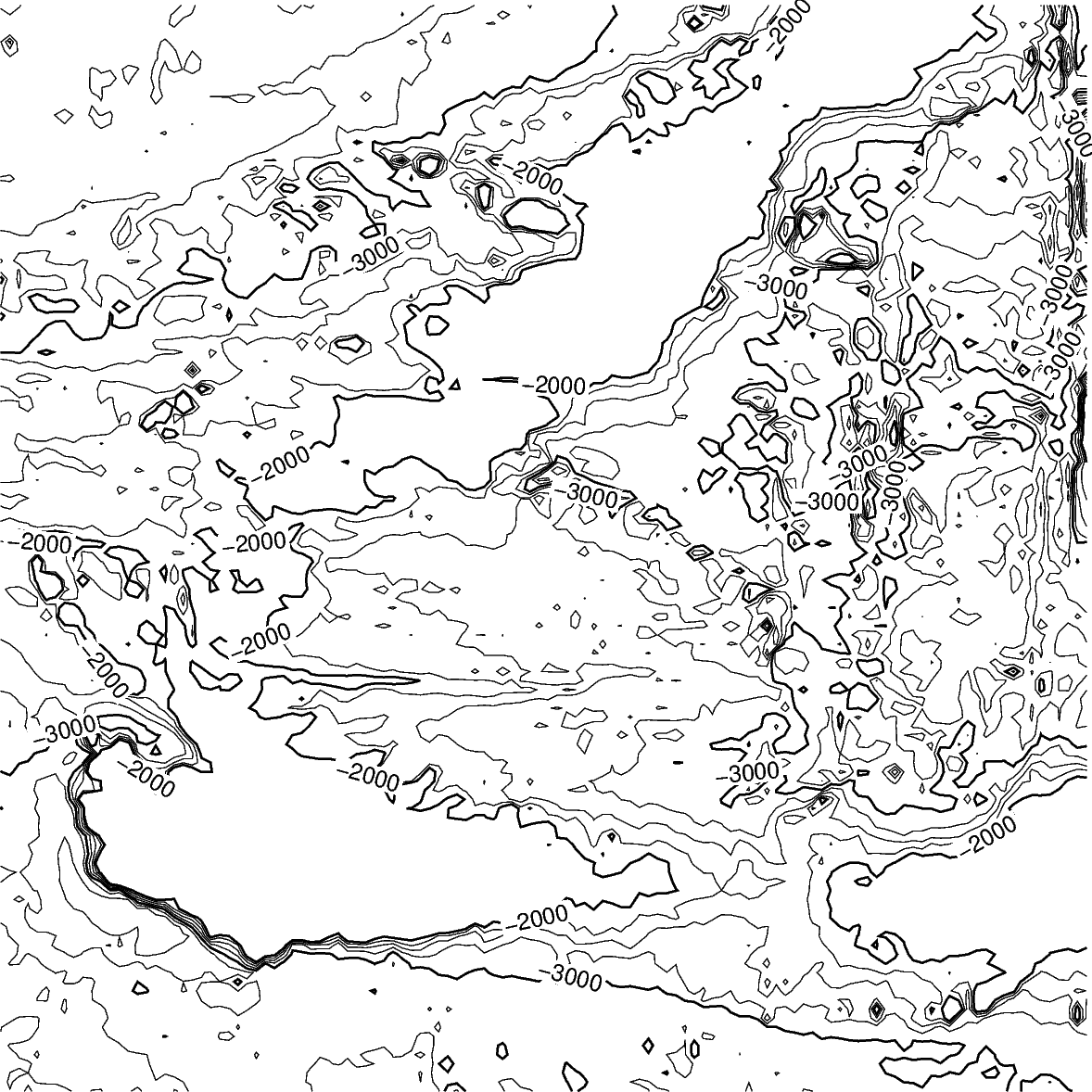Out:

<IPython.core.display.Image object>

Map settings

The pygmt.Figure.grdcontour method accepts additional arguments, including setting the projection and frame.

fig = pygmt.Figure()
fig.grdcontour(
annotation=1000,
interval=250,
grid=grid,
limit=[-4000, -2000],
projection="M10c",
frame=True,
)
fig.show()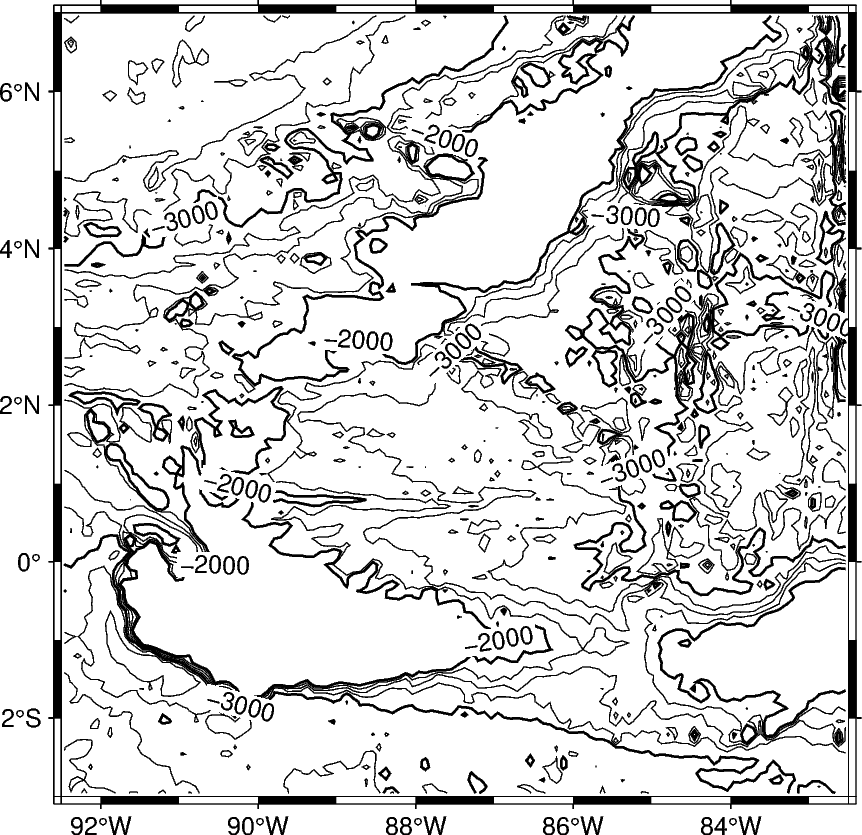Out:

<IPython.core.display.Image object>

The pygmt.Figure.grdimage method can be used to add a colormap to the contour map. It must be called prior to pygmt.Figure.grdcontour to keep the contour lines visible on the final map. If the projection argument is specified in the pygmt.Figure.grdimage method, it does not need to be repeated in the pygmt.Figure.grdcontour method.

fig = pygmt.Figure()
fig.grdimage(
grid=grid,
cmap="haxby",
projection="M10c",
frame=True,
)
fig.grdcontour(
annotation=1000,
interval=250,
grid=grid,
limit=[-4000, -2000],
)
fig.show()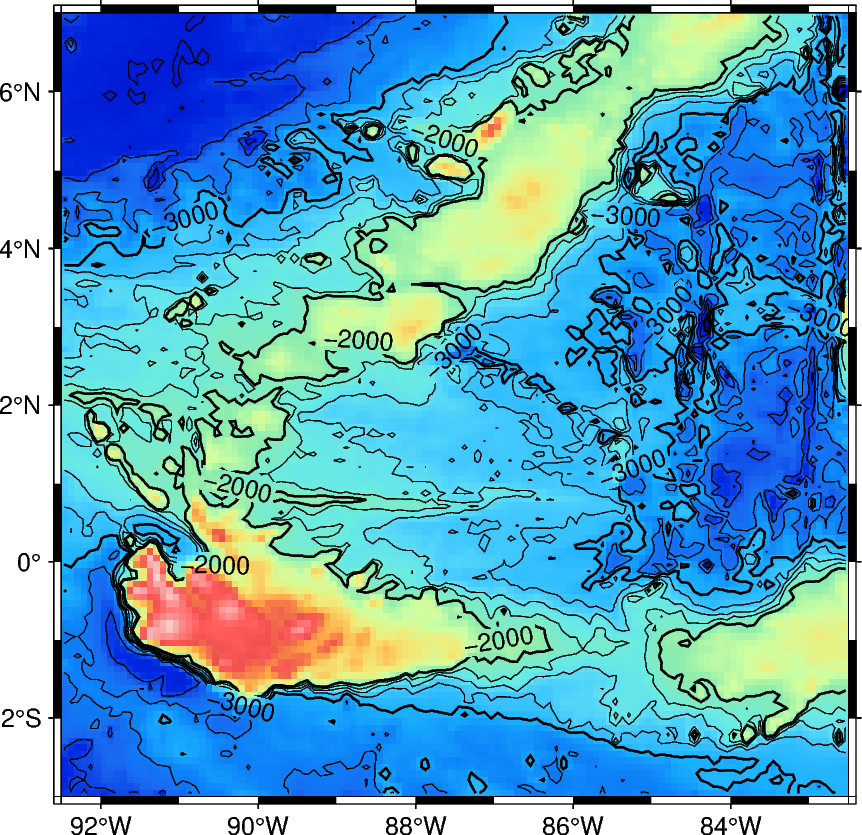Out:

<IPython.core.display.Image object>

Total running time of the script: ( 0 minutes 6.221 seconds)

Gallery generated by Sphinx-Gallery